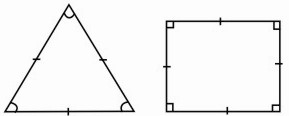HOME MATH DICTIONARY DOWNLOAD FEEDBACK DISCLAIMER
 Question: What is Regular Polygon ? Answer: A regular polygon is a polygon which has all its sides of equal length and all its angles equal. The simplest examples are an equilateral triangle and a square. A regular polygon with n sides can be easily drawn by starting with a circle and then drawing in radii at angles of 360o ÷ n to each other. The vertices of the polygon are at the ends of radii. The drawings show a regular pentagon and regular hexagon produced in this way. A regular n-sided polygon can be constructed with compass and straightedge if and only if the odd prime factors of n are distinct Fermat primes. Example of a Regular Polygon: